Autocorrelation plots

Introduction

This document aims to test whether DNA copy number profiles built by resampling within the acnrpackage are “realistic”, i.e. whether they have a similar spatial autocorrelation structure as the original data.

library("acnr")
library("R.utils")

We focus on one data set of the package, and look at the sample with the highest cellularity in this data set:

dataSets <- listDataSets()
dataSet <- dataSets
print(dataSet)
##  "GSE13372_HCC1143"
tfs <- listTumorFractions(dataSet)
tf <- tfs
(tf)
##  1
region <- as.factor(dat$region) We focus here on total copy numbers: x <- dat$c
rm(dat)

Autocorrelation plots

lim <- c(0, 0.05)
res <- sapply(levels(region), function (rr){
xTrue <- x[which(region==rr)]
xResamp <- sample(xTrue)
mar <- c(2, 2, 1, 0)+0.2
par(mar=mar)
acf(xTrue, ylim=lim)
mtext(sprintf("Original data: %s", rr), side=3)
acf(xResamp, ylim=lim)
mtext(sprintf("Resampled data: %s", rr), side=3)
})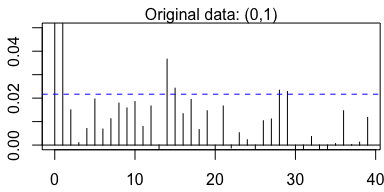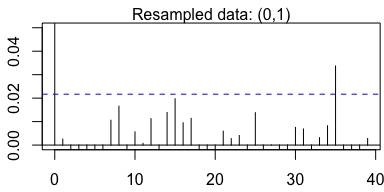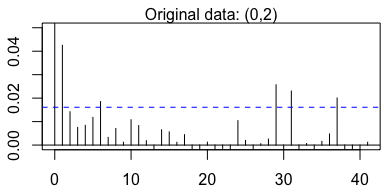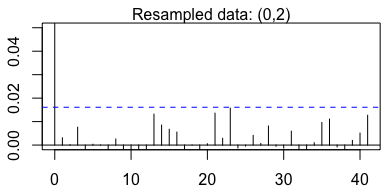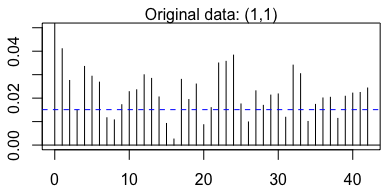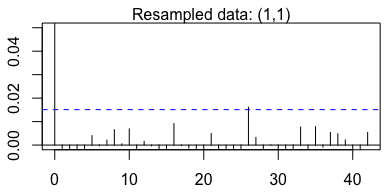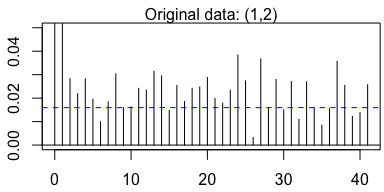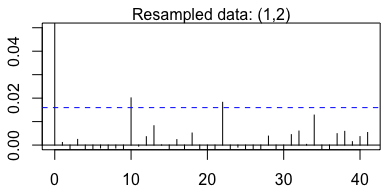From the autocorrelation plots it seems that the original data are slightly more autocorrelated than the resampled data. In order to check this hypothesis more quantatively, we perform the Ljung-Box test of the null hypothesis

• H0: The data are independently distributed (i.e. the correlations in the population from which the sample is taken are 0, so that any observed correlations in the data result from randomness of the sampling process).

against the alternative hypothesis

• H1: The data are not independently distributed; they exhibit serial correlation

Autocorrelation tests

tst <- "Ljung-Box"
lag <- 20
res <- sapply(levels(region), function (rr){
xTrue <- x[which(region==rr)]
len <- length(xTrue)
xResamp <- sample(xTrue)
bt <- Box.test(xTrue, lag=lag, type=tst)
br <- Box.test(xResamp, lag=lag, type=tst)
c("Original data"=bt$p.value, "Resampled data"=br$p.value, "Region size"=len)
})
cpt <- sprintf("$p$-values of the %s auto-correlation test (lag=%s)", tst, lag)
knitr::kable(t(res), caption=cpt)
$$p$$-values of the Ljung-Box auto-correlation test (lag=20)
Original data Resampled data Region size
(0,1) 1.00e-07 0.5510981 8175
(0,2) 6.75e-04 0.6528330 14798
(1,1) 0.00e+00 0.4384530 16856
(1,2) 0.00e+00 0.7561250 15087
plot(sort(res[2, ]), ylab="p-value", xlab="rank", main="sorted p-values", pch=19, col=3, ylim=c(0,1))
points(sort(res[1, ]), pch=19, col=1)
abline(a=0, b=1/ncol(res), lty=2)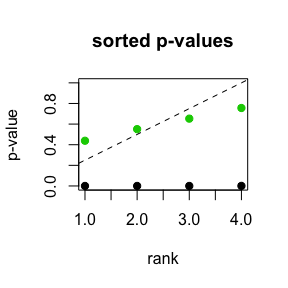The original data is clearly more autocorrelated than the resampled data. Most of the tests reject the null hypothesis for the original data, while they are not rejected on the resampled data. This implies that there exists a spatial correlation in the original data, and perfoming resampling breaks this correlation.

However, we note that this auto-correlation is sufficently weak not to be detected by the Ljung-Box test when applied on “only” 2,000 data points per region:

tst <- "Ljung-Box"
lag <- 20
maxSize <- 2000
res2 <- sapply(levels(region), function (rr){
len <- length(xTrue)
xResamp <- sample(xTrue)
bt <- Box.test(xTrue, lag=lag, type=tst)
br <- Box.test(xResamp, lag=lag, type=tst)
c("Original data"=bt$p.value, "Resampled data"=br$p.value, "Region size"=len)
})
cpt <- sprintf("$p$-values of the %s auto-correlation test (lag=%s) for regions of size <= %s", tst, lag, maxSize)
knitr::kable(t(res2), caption=cpt)
$$p$$-values of the Ljung-Box auto-correlation test (lag=20) for regions of size <= 2000
Original data Resampled data Region size
(0,1) 0.2124001 0.1918457 2000
(0,2) 0.4166288 0.7549016 2000
(1,1) 0.8984522 0.8876064 2000
(1,2) 0.8605090 0.9643987 2000
plot(sort(res2[2, ]), ylab="p-value", xlab="rank", main="sorted p-values", pch=19, col=3, ylim=c(0,1))
points(sort(res2[1, ]), pch=19, col=1)
abline(a=0, b=1/ncol(res), lty=2)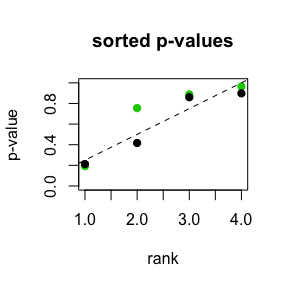Discussion

The original data is autocorrelated. This implies that the uniform resampling performed in the jointseg package yields copy number profiles whose signal distribution within regions of constant copy number level does not completely fit with the distribution of the original data. To address this issue, one possibility could be to perform block resampling. However, this raises the additional issue of chosing a block size.

We note that this autocorrelation is hardly detectable even in regions of 1,000 or 2,000 data points. Given the resolution of the Affymetrix GenomeWide SNP 6.0 chip type (on average, one data point every 1.5kb), this implies that the distribution of total copy numbers in regions smaller than a few megabases does provide a good approximation of the true distribution.

Session information

sessionInfo()
## R version 3.3.2 RC (2016-10-26 r71594)
## Platform: x86_64-apple-darwin13.4.0 (64-bit)
## Running under: OS X El Capitan 10.11.6
##
## locale:
##  C/fr_FR.UTF-8/fr_FR.UTF-8/C/fr_FR.UTF-8/fr_FR.UTF-8
##
## attached base packages:
##  stats     graphics  grDevices utils     datasets  methods   base
##
## other attached packages:
##  R.utils_2.5.0     R.oo_1.21.0       R.methodsS3_1.7.1 acnr_1.0.0
##  knitr_1.15.1
##
## loaded via a namespace (and not attached):
##   Rcpp_0.12.8     digest_0.6.12   rprojroot_1.1   backports_1.0.4
##   magrittr_1.5    evaluate_0.10   highr_0.6       stringi_1.1.2
##   rmarkdown_1.2   tools_3.3.2     stringr_1.1.0   yaml_2.1.14
##  htmltools_0.3.5